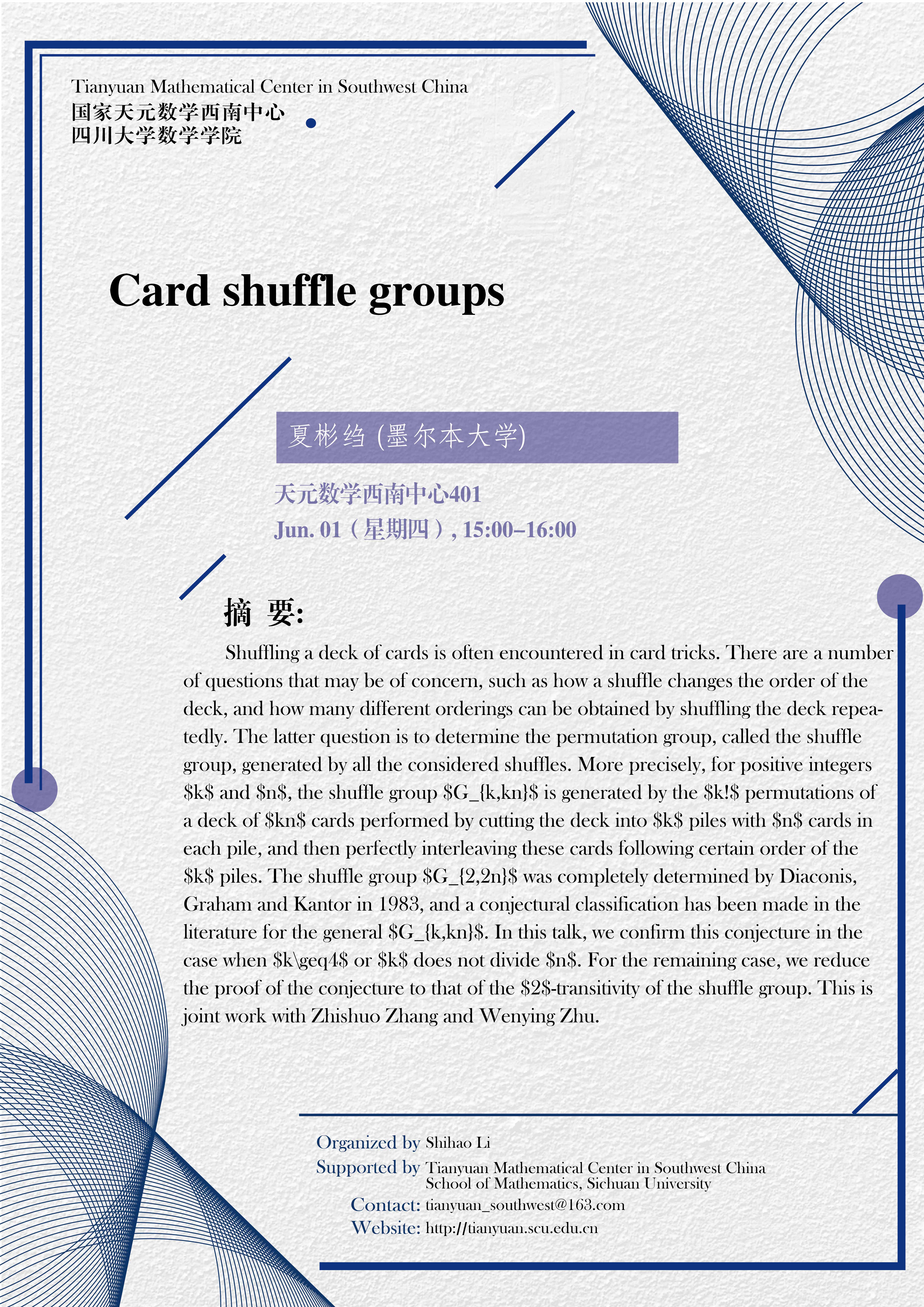Card shuffle groups

Shuffling a deck of cards is often encountered in card tricks. There are a number of questions that may be of concern, such as how a shuffle changes the order of the deck, and how many different orderings can be obtained by shuffling the deck repeatedly. The latter question is to determine the permutation group, called the shuffle group, generated by all the considered shuffles. More precisely, for positive integers \$k\$ and \$n\$, the shuffle group \$G_{k,kn}\$ is generated by the \$k!\$ permutations of a deck of \$kn\$ cards performed by cutting the deck into \$k\$ piles with \$n\$ cards in each pile, and then perfectly interleaving these cards following certain order of the \$k\$ piles. The shuffle group \$G_{2,2n}\$ was completely determined by Diaconis, Graham and Kantor in 1983, and a conjectural classification has been made in the literature for the general \$G_{k,kn}\$. In this talk, we confirm this conjecture in the case when \$k\geq4\$ or \$k\$ does not divide \$n\$. For the remaining case, we reduce the proof of the conjecture to that of the \$2\$-transitivity of the shuffle group. This is joint work with Zhishuo Zhang and Wenying Zhu.